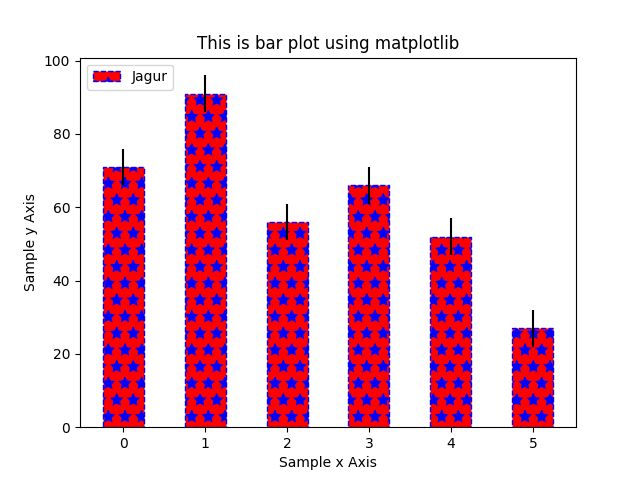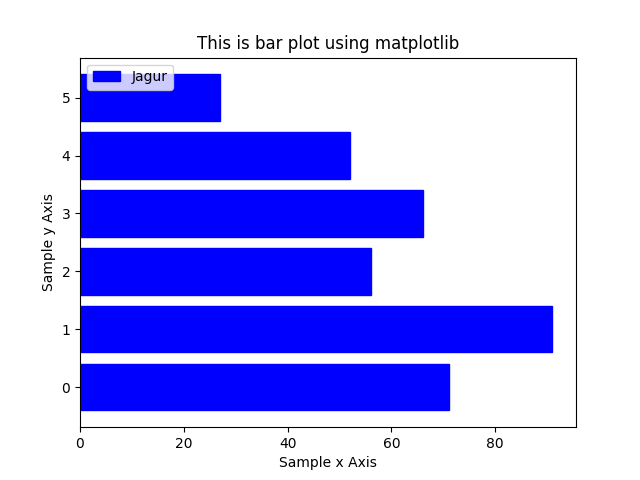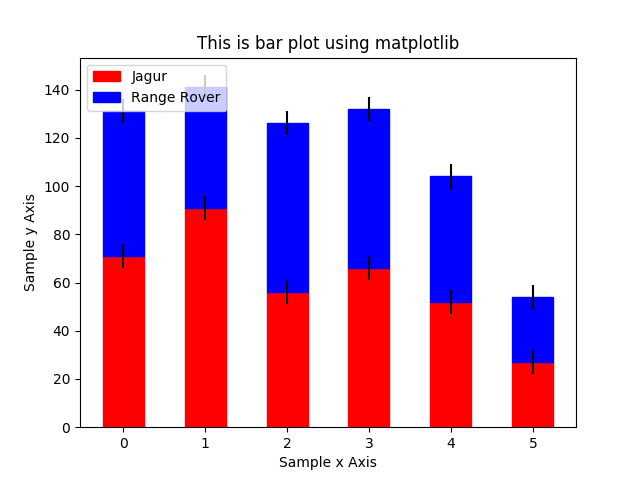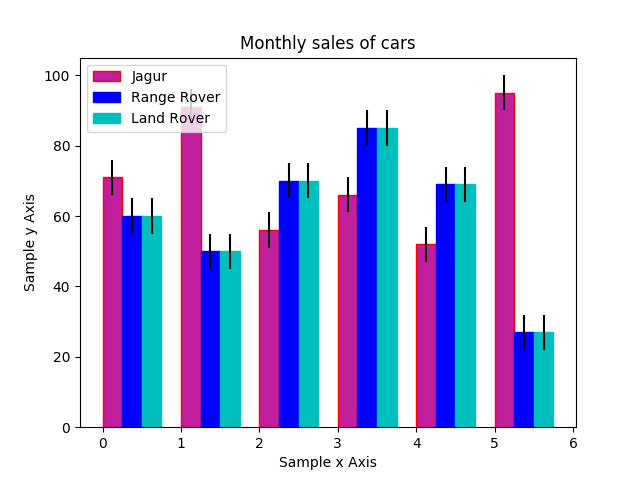# Bar plots in Matplotlib – Data Visualization using Python

Matplotlib can be used to draw simple as well as complex bar plots. In this article we will see how to draw bar plots along with available options.

Here is an example of simple bar plot with available options.

Output of this scriptHorizontal Bar plot

OutputStacked bar plot

Outputbar plot with multiple data series